Question

The tree diagram below depicts a heap being used to implement a priority queue. Enqueue the value 6 onto the priority queue, then execute a dequeue. After those two operations have been completed, what does the underlying array look like? List the elements in the resulting heap in the order in which they appear in the array, from left to right.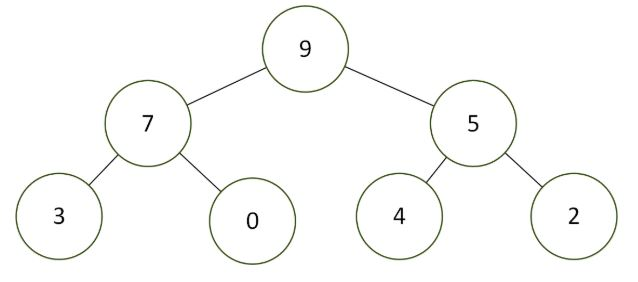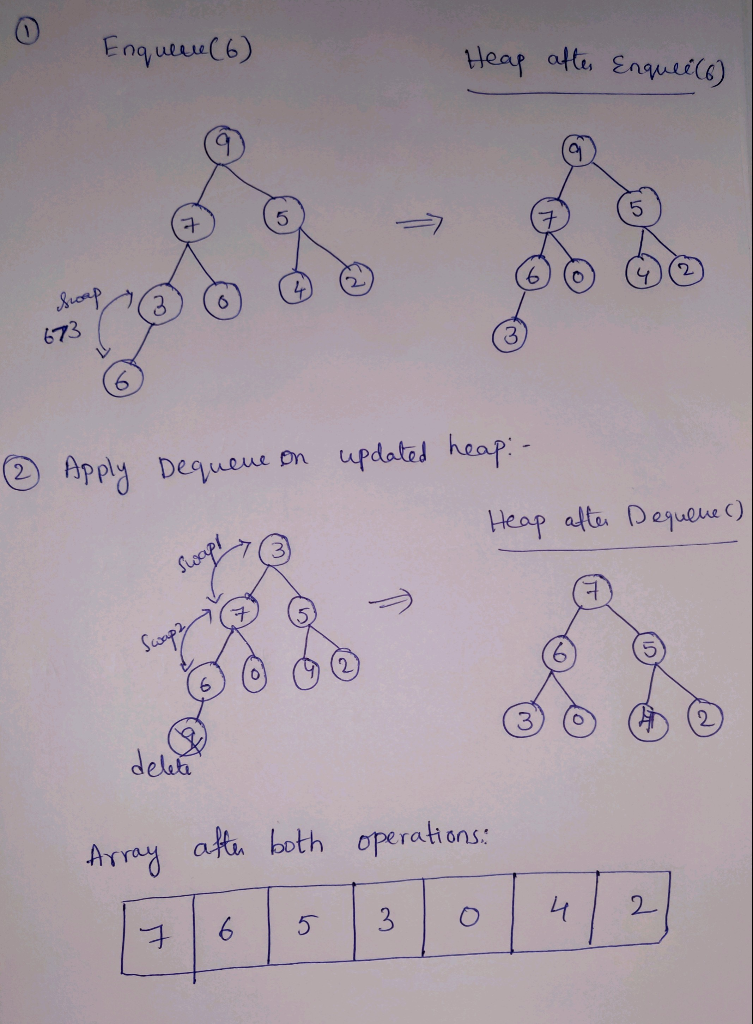#### Earn Coins

Coins can be redeemed for fabulous gifts.

Similar Homework Help Questions
• ### Suppose we want to implement a circular queue using an array that has an initial capacity...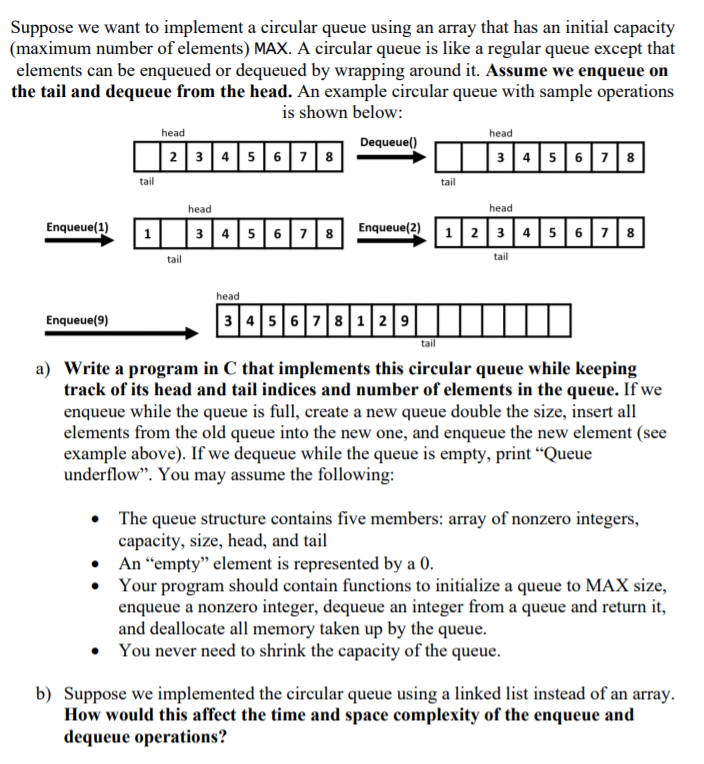Suppose we want to implement a circular queue using an array that has an initial capacity (maximum number of elements) MAX. A circular queue is like a regular queue except that elements can be enqueued or dequeued by wrapping around it. Assume we enqueue on the tail and dequeue from the head. An example circular queue with sample operations is shown below: head head tail head tail tail head Enqueue(9) a) Write a program in C that implements this circular...

• ### In class, we discussed the priority queue (PQ) ADT implemented using min-heap. In a min-heap, the...In class, we discussed the priority queue (PQ) ADT implemented using min-heap. In a min-heap, the element of the heap with the smallest key is the root of the binary tree. On the other hand, a max-heap has as root the element with the biggest key, and the relationship between the keys of a node and its parent is reversed of that of a min-heap. We also discussed an array-based implementation of heaps. In this assignment, your task is to...

• ### Write a program in Java to implement the max-priority queue using max-heap data structure. Implement the...

Write a program in Java to implement the max-priority queue using max-heap data structure. Implement the max-heap data structure using an integer array of 10 cells. (Do not use Java in-built PriorityQueue class.) [In a max-heap, the root node and the intermediate node vales are always greater than their children.] First, take 10 integer values from the user and insert them in the max-priority queue. Then print the elements of the queue. After that, delete two elements from the queue...

• ### solving using C. Use a singly linked list to implement a priority queue with two operations:...

solving using C. Use a singly linked list to implement a priority queue with two operations: enqueue and dequeue. Each node contains an integer value, a priority, and a pointer to the next node. The priority is a value between 1- 10 (where 10 is the highest priority). When a value is added to the queue, it is added with a value and priority. When a value is removed from the priority queue, the first element with the highest priority...

• ### I am to implement a simple simulation that supports a stack and a queue of items being either enqueued and dequeued (ont...

I am to implement a simple simulation that supports a stack and a queue of items being either enqueued and dequeued (onto the queue) or pushed and popped (onto the stack). I are required to use STL data structures to implement and create the stack and queue for my program. ----- testfile1.tst -------- enqueue 5 enqueue 7 push blooper push rookie dequeue push demerits pop enqueue 3 enqueue 8 push showplace enqueue 9 dequeue pop dequeue push palmetto push zebra...

• ### e. public class Queue { // Uses the correct Stack class from ME2 Ex 19 private Stack mStack; public Queue() { setStack(new Stack()); } public Queue enqueue(E pData) { getStack().push(pData); return t...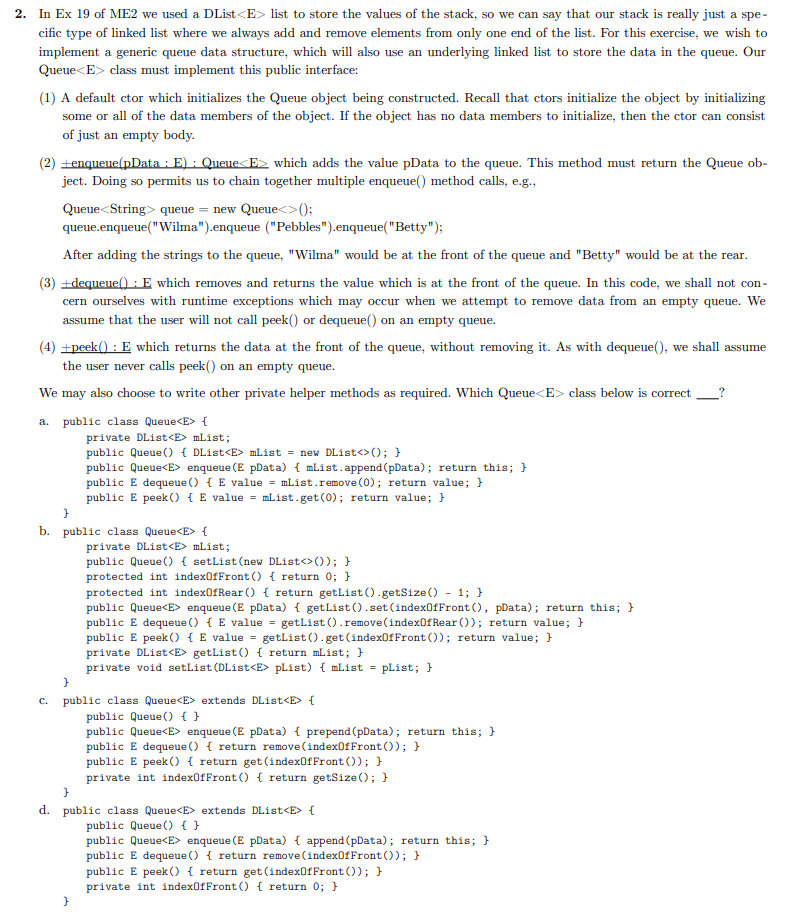e. public class Queue { // Uses the correct Stack class from ME2 Ex 19 private Stack mStack; public Queue() { setStack(new Stack()); } public Queue enqueue(E pData) { getStack().push(pData); return this; } public E dequeue() { return getStack().pop(); } public E peek() { return getStack.peek(); } private Stack getStack() { return mStack; } private void setStack(Stack pStack) { mStack = pStack; } } f. public class Queue extends Stack { // Uses the correct Stack class from ME2 Ex...

• ### 5. A three-heap with n elements can be stored in an array A, where A[O] contains...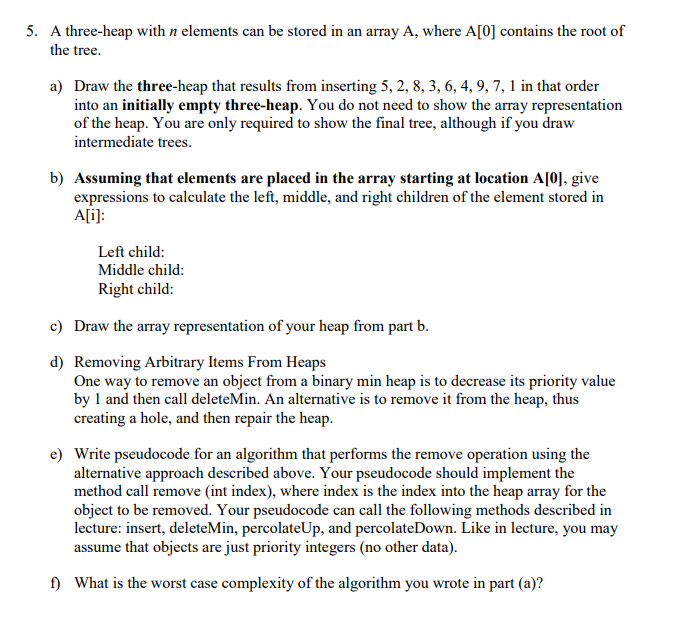5. A three-heap with n elements can be stored in an array A, where A[O] contains the root of the tree. a) Draw the three-heap that results from inserting 5, 2, 8, 3, 6, 4, 9, 7, 1 in that order into an initially empty three-heap. You do not need to show the array representation of the heap. You are only required to show the final tree, although if you draw intermediate trees. b) Assuming that elements are placed in...

• ### A priority queue is a collection of items each having a priority. A priority queue supports three...

A priority queue is a collection of items each having a priority. A priority queue supports three fundamental operations. You can ask a priority queue whether it is empty. You can insert an item into the priority queue with a given priority. You can remove the item from the priority queue that has the smallest priority. For example, suppose that you start with an empty priority queue and imagine performing the following steps. Insert item "one" with priority 10. Insert...

• ### JAVA LANG PLEASE: I have follwed these below guidelines but when i run my queue test...

JAVA LANG PLEASE: I have follwed these below guidelines but when i run my queue test it is not executing but my stack is working fine, can you fix it please! MyQueue.java Implement a queue using the MyStack.java implementation as your data structure.  In other words, your instance variable to hold the queue items will be a MyStack class. enqueue(String item): inserts item into the queue dequeue(): returns and deletes the first element in the queue isEmpty(): returns true or false...

• ### In Java. How would this method look? LinkedBinaryTree.java import java.util.Iterator; public class LinkedBinaryTree implements BinaryTreeADT {...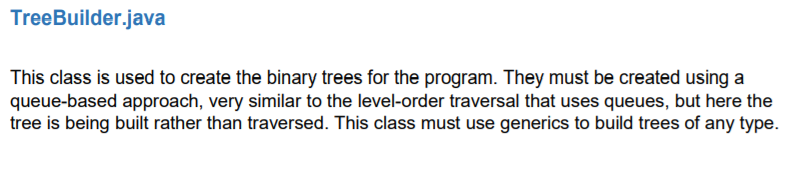In Java. How would this method look? LinkedBinaryTree.java import java.util.Iterator; public class LinkedBinaryTree implements BinaryTreeADT {    private BinaryTreeNode root;    /**    * Creates an empty binary tree.    */    public LinkedBinaryTree() {        root = null;    }    /**    * Creates a binary tree from an existing root.    */    public LinkedBinaryTree(BinaryTreeNode root) {        this.root = root;    }    /**    * Creates a binary tree with the specified element...